# Pie and Doughnut in WinUI Chart

27 May 202215 minutes to read

## Pie

`PieSeries` is divided into sectors, illustrating numerical proportion. The following code example illustrates the PieSeries.

``<chart:PieSeries XBindingPath="Category" ItemsSource="{Binding Data}" YBindingPath="Value"/>``
``````PieSeries series = new PieSeries()
{

ItemsSource = new ViewModel().Data,

XBindingPath = "Category",

YBindingPath = "Value"

};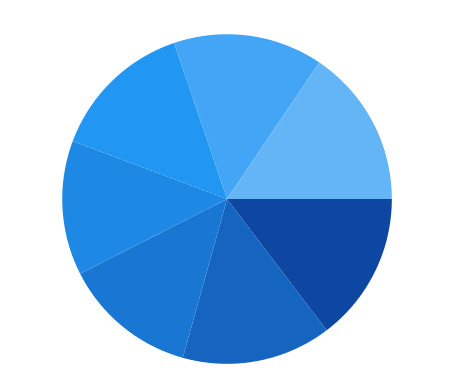The rendering size of the PieSeries can be controlled using the `CircularCoefficient` property, as shown in the following code example.

``<chart:PieSeries CircularCoefficient="0.9" XBindingPath="Category" ItemsSource="{Binding Data}" YBindingPath="Value"/>``
``````PieSeries series = new PieSeries()
{

ItemsSource = new ViewModel().Data,

XBindingPath = "Category",

YBindingPath = "Value",

CircularCoefficient = 0.9

};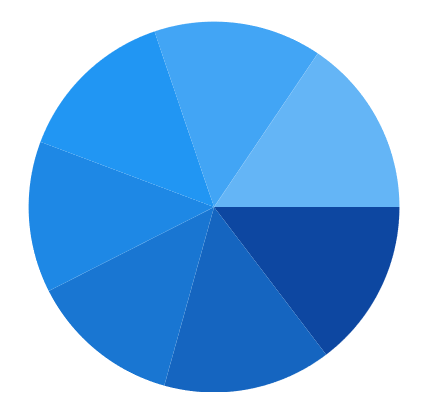### Group small data points into “others”

The small segments in the pie chart can be grouped into the “others” category using the `GroupTo` and `GroupMode` properties of PieSeries.

The `GroupMode` property is used to specify the grouping type based on slice `Angle`, actual data point `Value`, or `Percentage`, and the `GroupTo` property is used to set the limit to group data points into a single slice. The grouped segment is labeled as “Others” in the legend and toggled as other segment. The default value of the `GroupTo` and `GroupMode` properties is `double.NAN` and `Value.`

Pie series without grouping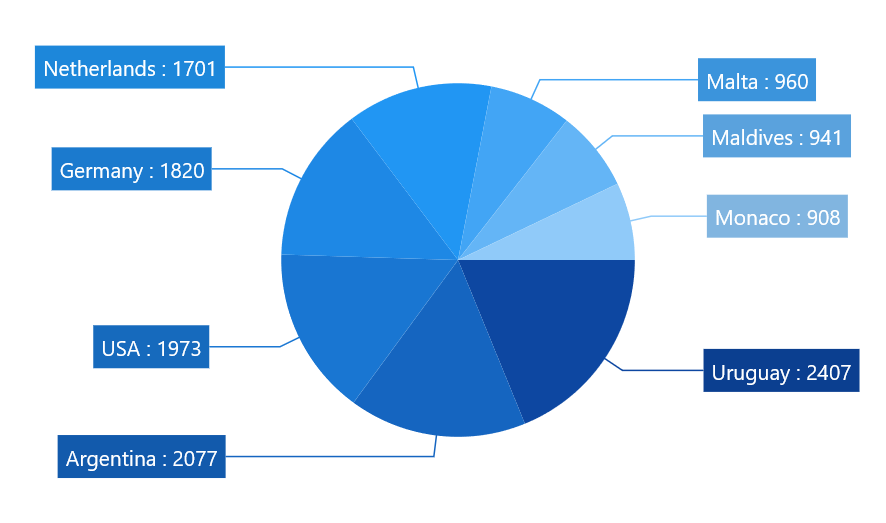Pie series with grouping (Mode - Value)

``````<chart:PieSeries ItemsSource="{Binding Data}" XBindingPath="Country" YBindingPath="Count" GroupMode="Value" GroupTo="1000">
<chart:PieSeries.DataMarker>
<chart:ChartDataMarker ShowConnectorLine="True"
ConnectorHeight="80"
ShowLabel="True"
LabelTemplate="{StaticResource DataLabelTemplate}"
SegmentLabelContent="LabelContentPath">
</chart:ChartDataMarker>
</chart:PieSeries.DataMarker>
</chart:PieSeries>``````
``````pieSeries.GroupMode = PieGroupMode.Value;
pieSeries.GroupTo = 1000;
ChartDataMarker datamarker = new ChartDataMarker()
{
ShowConnectorLine = true,
ShowLabel = true,
ConnectorHeight = 80,
LabelTemplate = this.RootGrid.Resources["DataLabelTemplate"] as DataTemplate,
SegmentLabelContent = LabelContent.LabelContentPath,

pieSeries.DataMarker = datamarker;``````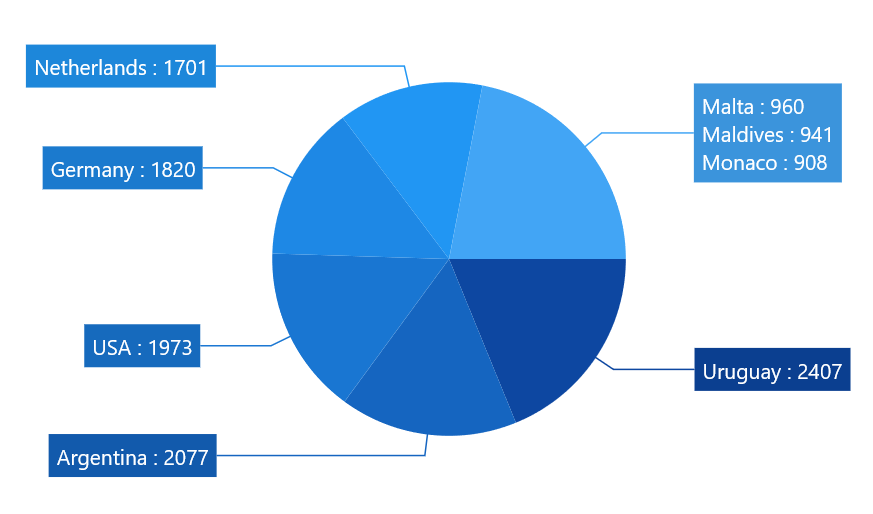Pie series with grouping (Mode - Angle)

``````<chart:PieSeries ItemsSource="{Binding Data}" XBindingPath="Country" YBindingPath="Count" GroupMode="Angle" GroupTo="30">
<chart:PieSeries.DataMarker>
<chart:ChartDataMarker ShowConnectorLine="True"
ConnectorHeight="80"
ShowLabel="True"
LabelTemplate="{StaticResource DataLabelTemplate}"
SegmentLabelContent="LabelContentPath">
</chart:ChartDataMarker>
</chart:PieSeries.DataMarker>
</chart:PieSeries>``````
``````pieSeries.GroupMode = PieGroupMode.Angle;
pieSeries.GroupTo = 30;

ChartDataMarker datamarker = new ChartDataMarker()
{
ShowConnectorLine = true,
ShowLabel = true,
ConnectorHeight = 80,
LabelTemplate = this.RootGrid.Resources["DataLabelTemplate"] as DataTemplate,
SegmentLabelContent = LabelContent.LabelContentPath,
};

pieSeries.DataMarker = datamarker;``````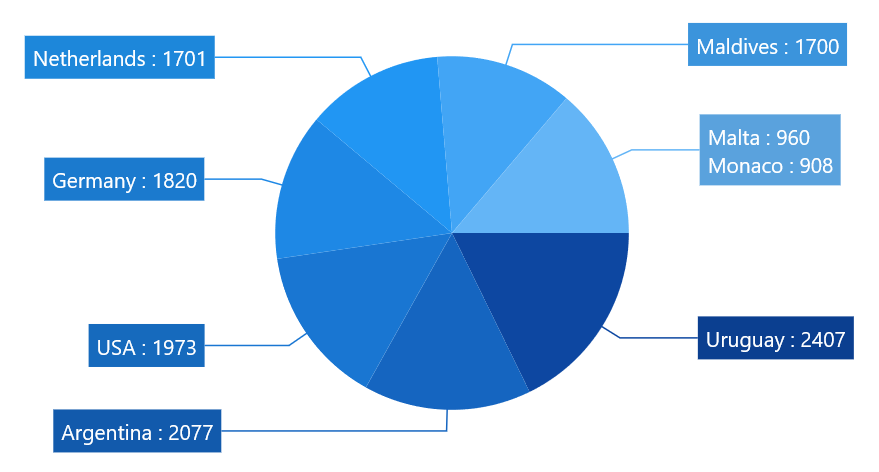Pie series with grouping (Mode - Percentage)

``````<chart:PieSeries ItemsSource="{Binding Data}" XBindingPath="Country" YBindingPath="Count" GroupMode="Percentage" GroupTo="10">
<chart:PieSeries.DataMarker>
<chart:ChartDataMarker ShowConnectorLine="True"
ConnectorHeight="80"
ShowLabel="True"
LabelTemplate="{StaticResource DataLabelTemplate}"
SegmentLabelContent="LabelContentPath">
</chart:ChartDataMarker>
</chart:PieSeries.DataMarker>
</chart:PieSeries>``````
``````pieSeries.GroupMode = PieGroupMode.Percentage;
pieSeries.GroupTo = 10;

ChartDataMarker datamarker = new ChartDataMarker()
{
ShowConnectorLine = true,
ShowLabel = true,
ConnectorHeight = 80,
LabelTemplate = this.RootGrid.Resources["DataLabelTemplate"] as DataTemplate,
SegmentLabelContent = LabelContent.LabelContentPath,
};

pieSeries.DataMarker = datamarker;``````## Doughnut

`DoughnutSeries` is similar to PieSeries. It is used to show the relationship between parts of data and whole data.

The DoughnutSeries can be added to the chart, as shown in the following code example.

``<chart:DoughnutSeries XBindingPath="Category" ItemsSource="{Binding Data}" YBindingPath="Value"/>``
``````DoughnutSeries series = new DoughnutSeries()
{

ItemsSource = new ViewModel().Data,

XBindingPath = "Category",

YBindingPath = "Value"
};The Doughnut also has a `coefficient` property, `DoughnutCoefficient,` which defines the inner circle. Also, it has `DoughnutSize`, which is used to define the size for this series like `CircularCoefficient` in PieSeries.

``<chart:DoughnutSeries DoughnutCoefficient="0.7" XBindingPath="Category" ItemsSource="{Binding Data}" YBindingPath="Value"/>``
``````DoughnutSeries series = new DoughnutSeries()
{

ItemsSource = new ViewModel().Data,

XBindingPath = "Category",

YBindingPath = "Value",

DoughnutCoefficient = 0.7
};Size

The size of doughnut series can be customized by using the `DoughnutSize` property. The following code illustrates how to use the property in series.

``````<syncfusion:SfChart>

<syncfusion:DoughnutSeries DoughnutSize="0.8"/>

<syncfusion:DoughnutSeries DoughnutSize="0.8"/>

</syncfusion:SfChart>``````
``````DoughnutSeries doughnut = new DoughnutSeries();

doughnut.DoughnutSize = 0.8;

DoughnutSeries doughnut1 = new DoughnutSeries();

doughnut1.DoughnutSize = 0.8;Hole Size

`DoughnutHoleSize` is an `attachable` property. It gets or sets the double value, which is used to customize the doughnut hole size. Its value ranges from 0 to 1, and it can be set as shown in the following code example.

``````<Chart:SfChart Chart:DoughnutSeries.DoughnutHoleSize="0.2">

</chart:SfChart>``````
``````SfChart chart = new SfChart();

DoughnutSeries.SetDoughnutHoleSize(chart, 0.2);``````## Semi Pie and Doughnut

By using the custom `StartAngle` and `EndAngle` properties, you can draw pie series in different shapes such as semicircular or quarter circular series.

``<syncfusion:PieSeries StartAngle="180" EndAngle="360" XBindingPath="Utilization" YBindingPath="ResponseTime" ItemsSource="{Binding Data}"/>``
``````PieSeries series = new PieSeries()
{

ItemsSource = new ViewModel().Data,

XBindingPath = "Utilization",

YBindingPath = "ResponseTime",

StartAngle = 180,

EndAngle = 360

};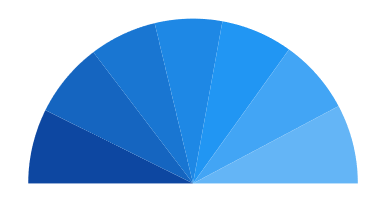``<syncfusion:DoughnutSeries StartAngle="180" EndAngle="360" XBindingPath="Utilization" YBindingPath="ResponseTime" ItemsSource="{Binding Data}"/>``
``````DoughnutSeries series = new DoughnutSeries()
{

ItemsSource = new ViewModel().Data,

XBindingPath = "Utilization",

YBindingPath = "ResponseTime",

StartAngle = 180,

EndAngle = 360

};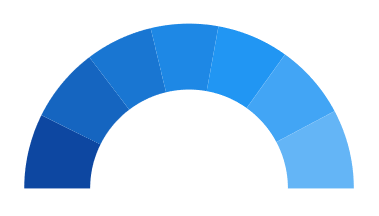## Stacked doughnut

Doughnut segments can be separated as individual circles using the `IsStackedDoughnut` property. The following properties are used to customize the stacked doughnut chart:

``````<chart:SfChart>
…
<chart:DoughnutSeries IsStackedDoughnut="True" CapStyle="BothCurve" SegmentSpacing="0.2" MaximumValue="100" XBindingPath="Category" YBindingPath="Expenditure" ItemsSource="{Binding ExpenditureData}">
</chart:DoughnutSeries>

</chart:SfChart>``````
``````SfChart chart = new SfChart();

DoughnutSeries doughnutSeries = new DoughnutSeries()
{
IsStackedDoughnut = true,
CapStyle = DoughnutCapStyle.BothCurve,
SegmentSpacing = 0.2,
MaximumValue = 100,
XBindingPath = "Category",
YBindingPath = "Expenditure",
ItemsSource = new ViewModel().ExpenditureData
};## Explode Segments

The following properties are used to explode the individual segments in Pie and Doughnut.

Explode Index

``````<syncfusion:PieSeries x:Name="PieSeries" ItemsSource="{Binding Data}"

YBindingPath="ResponseTime" />``````
``````PieSeries series = new PieSeries()
{

ItemsSource = new ViewModel().Data,

XBindingPath = "Utilization",

YBindingPath = "ResponseTime",

ExplodeIndex = 2,

};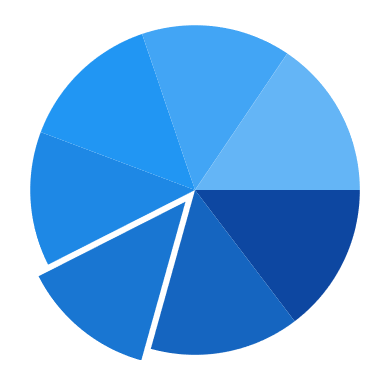NOTE

ExplodeRadius has been defined as 30, by default its value is zero. So you need to define explode, when you set ExplodeIndex or ExplodeAll.

Explode All

``````<chart:PieSeries  ExplodeAll="True" ExplodeRadius="15"

XBindingPath="Category" ItemsSource="{Binding Data}"

YBindingPath="Value">``````
``````PieSeries series = new PieSeries()
{

ItemsSource = new ViewModel().Data,

XBindingPath = "Category",

YBindingPath = "Value",

ExplodeAll = true,